# Exploratory Analysis II

Data Visualization, part 2. Code for Quiz 8

1. Load the packages we will use.
``````library(tidyverse)
library(patchwork)
``````
1. Quiz questions
• Replace all the ???s. These are answers on your moodle quiz.

• Run all the individual code chunks to make sure the answers in this file correspond with your quiz answers

• After you check all your code chunks run then you can knit it. It won’t knit until the ??? are replaced

• The quiz assumes that you have watched the videos, downloaded (to your examples folder) and worked through the exercises in exercises_slides-50-61.Rmd

• Pick one of your plots to save as your preview plot. Use the ggsave command at the end of the chunk of the plot that you want to preview.

# Question: modify slide 51

• Create a plot with the `mpg dataset`

• add points with `geom_point`

• assign the variable `displ` to the x-axis

• assign the variable `hwy` to the y-axis

• add `facet_wrap` to split the data into panels based on the `manufacturer`

``````ggplot(data = mpg) +
geom_point(aes(x = displ, y = hwy)) +
facet_wrap(facets = vars(manufacturer))
``````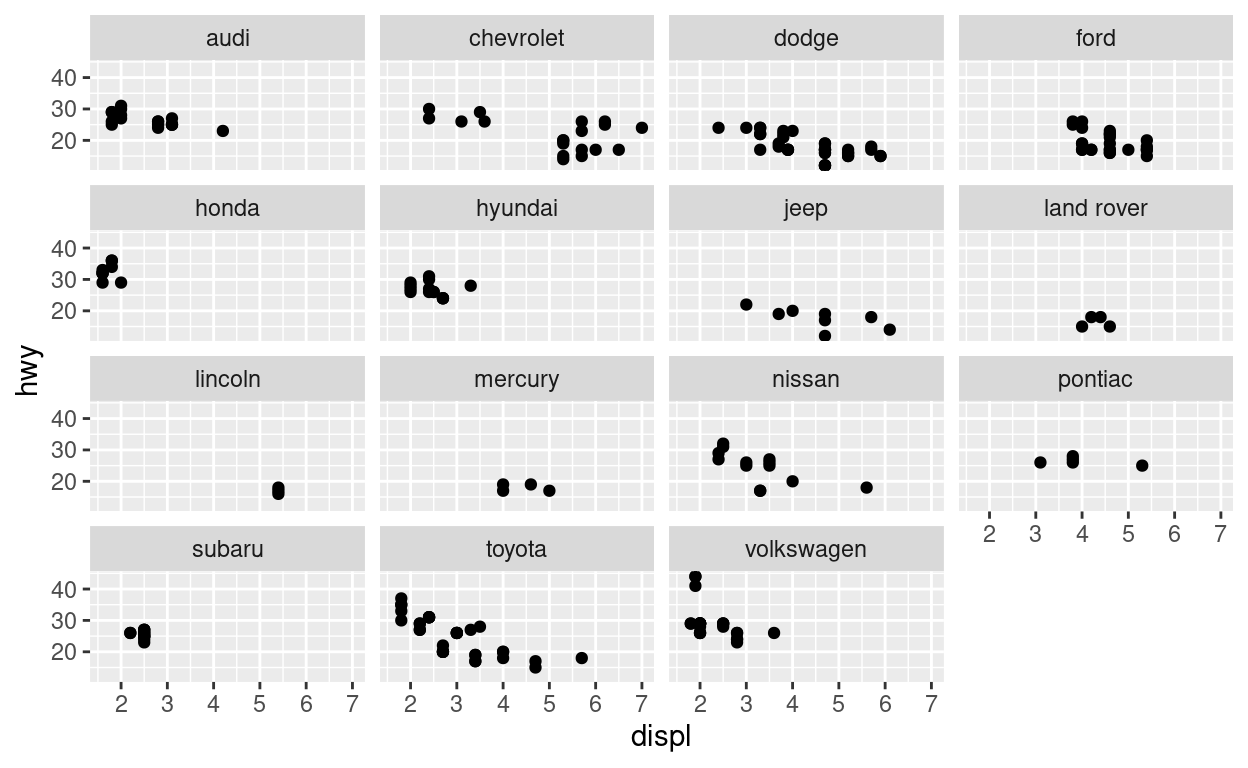# Question: modify facet-ex-2

• Create a plot with the `mpg dataset`

• add bars with with `geom_bar`

• assign the variable `manufacturer` to the y-axis

• add `facet_grid` to split the data into panels based on the `class`

• let scales vary across columns

• let space taken up by panels vary by columns

``````ggplot(mpg) +
geom_bar(aes(y = manufacturer)) +
facet_grid(vars(class), scales = "free_y", space = "free_y")
``````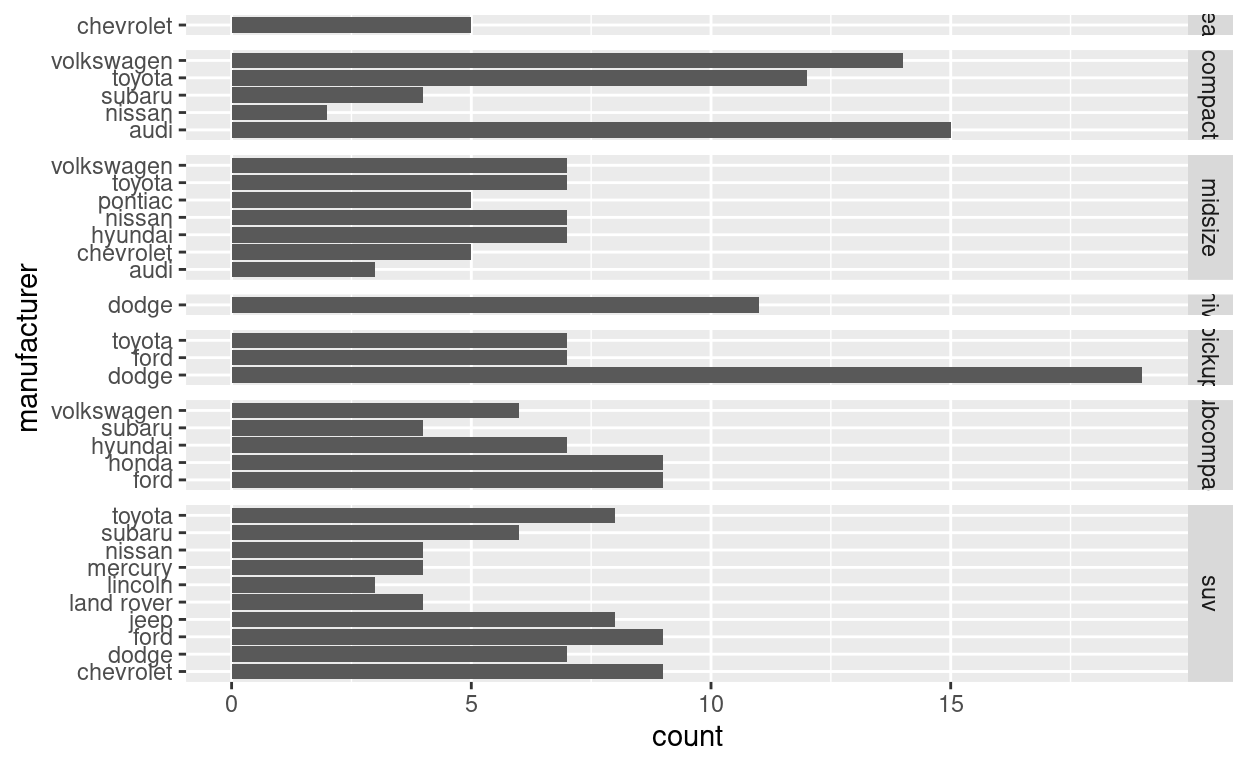# Question: spend_time

• the patchwork slides and

• `spend_time` contains 10 years of data on how many hours Americans spend each day on 5 activities

read it into `spend_time`

``````spend_time  <- read_csv("spend_time.csv")
``````

• extract observations for 2018

• THEN create a plot with that data

• ADD a barchart with with `geom_col`

– assign `activity` to the x-axis

– assign `avg_hours` to the y-axis

– assign `activity` to fill

• ADD `scale_y_continuous` with breaks every hour from 0 to 6 hours

• ADD `labs` to

– set `subtitle` to Avg hours per day: 2018

– set `x` and `y` to NULL so they won’t be labeled

• assign the output to `p1`

• display `p1`

``````p1  <- spend_time %>% filter(year == "2018")  %>%
ggplot() +
geom_col(aes(x = activity, y = avg_hours, fill = activity)) +
scale_y_continuous(breaks = seq(0, 6, by = 1)) +
labs(subtitle = "Avg hours per day: 2018", x = NULL, y = NULL)

p1
``````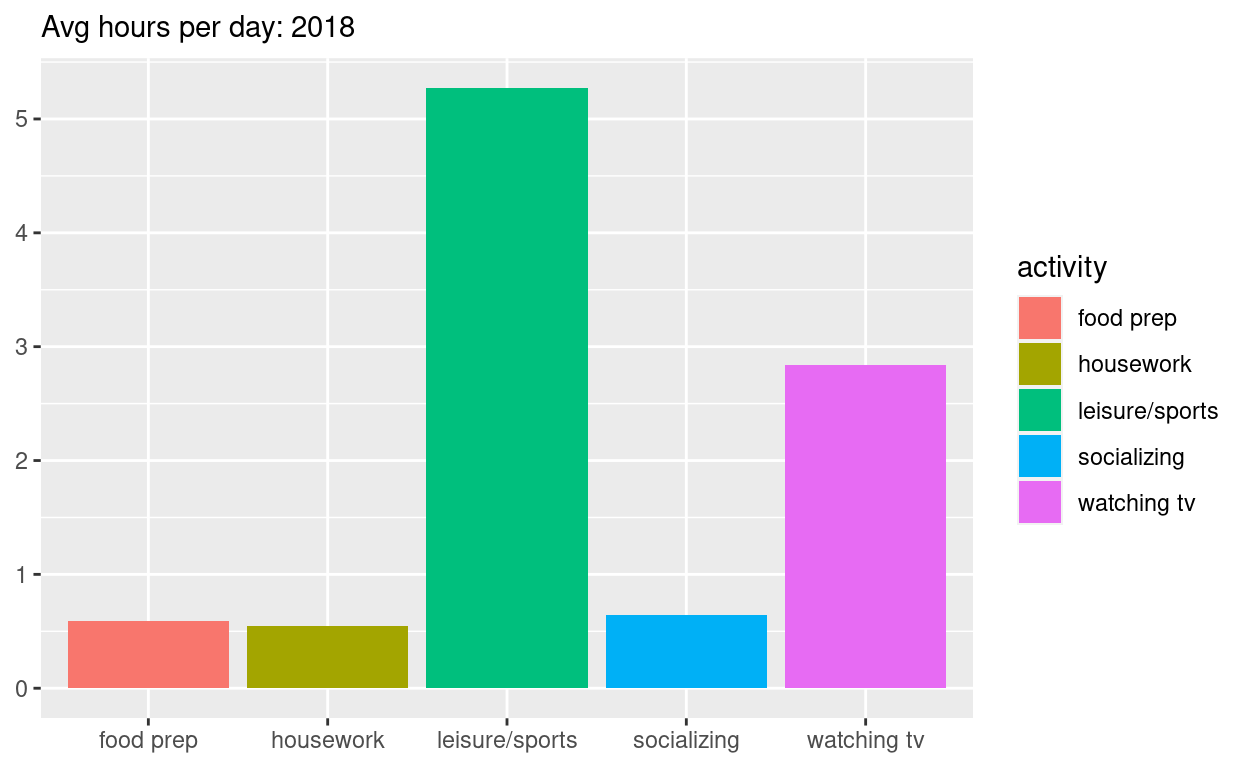• THEN create a plot with it

• ADD a barchart with with geom_col

-assign year to the x-axis

-assign avg_hours to the y-axis

-assign activity to fill

• set subtitle to “Avg hours per day: 2010-2019”

• set x and y to NULL so they won’t be labeled

• assign the output to p2

• display p2

``````p2  <- spend_time  %>%
ggplot() +
geom_col(aes(x = year, y = avg_hours, fill = activity)) +
labs(subtitle  = "Avg hours per day: 2010-2019", x = NULL, y = NULL)

p2
``````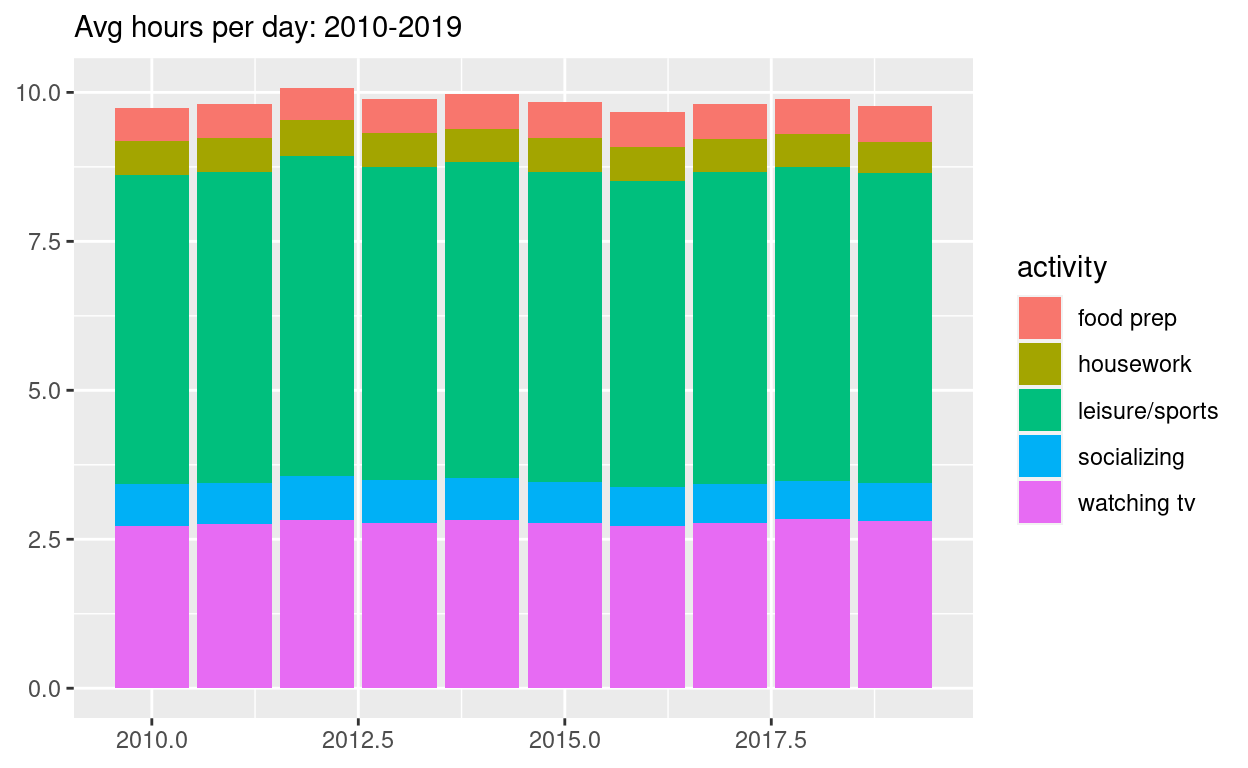``````ggsave(filename = "preview.png",
path = here::here("_posts", "2022-03-28-exploratory-analysis-ii"))
``````

• Use patchwork to display `p1` on top of `p2`

• assign the output to `p_all` `

• display `p_all`

``````p_all  <-  p1 / p2

p_all
``````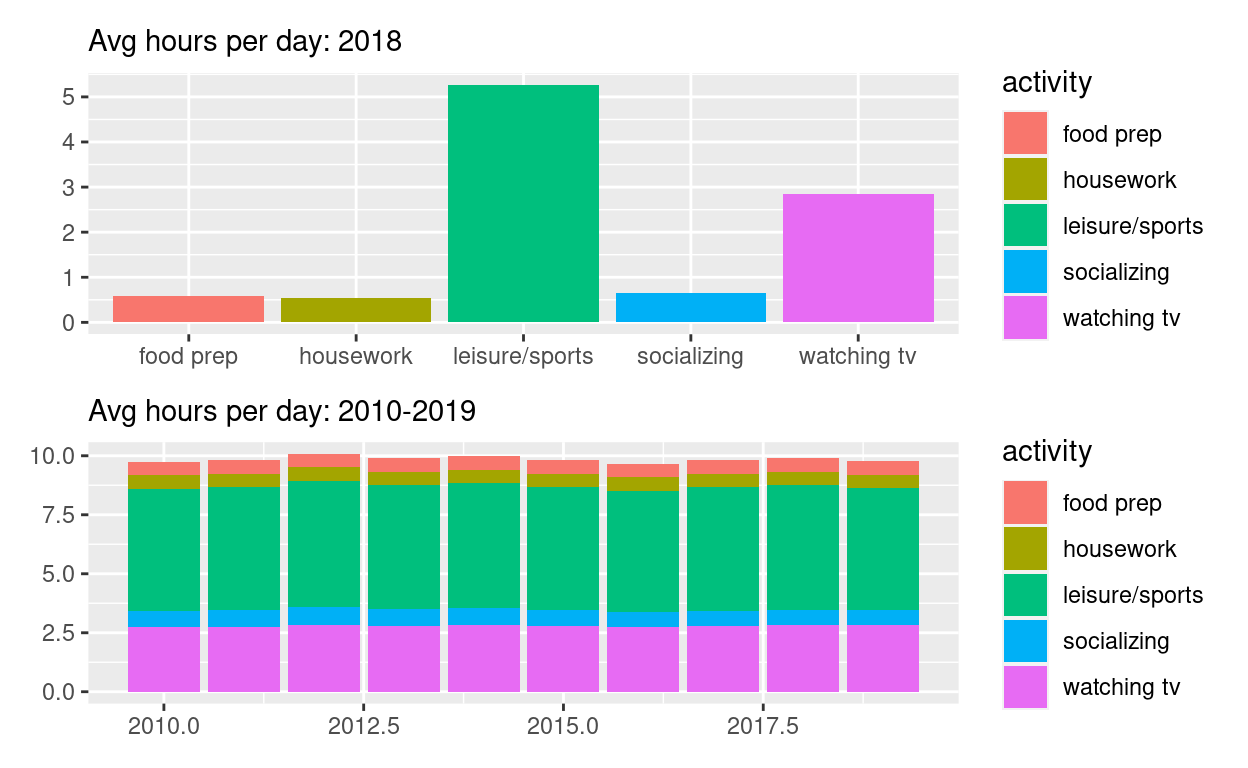• AND set `legend.position` to ‘none’ to get rid of the legend

• assign the output to `p_all_no_legend`

• display `p_all_no_legend`

``````p_all_no_legend  <- p_all & theme(legend.position = 'none')
p_all_no_legend
``````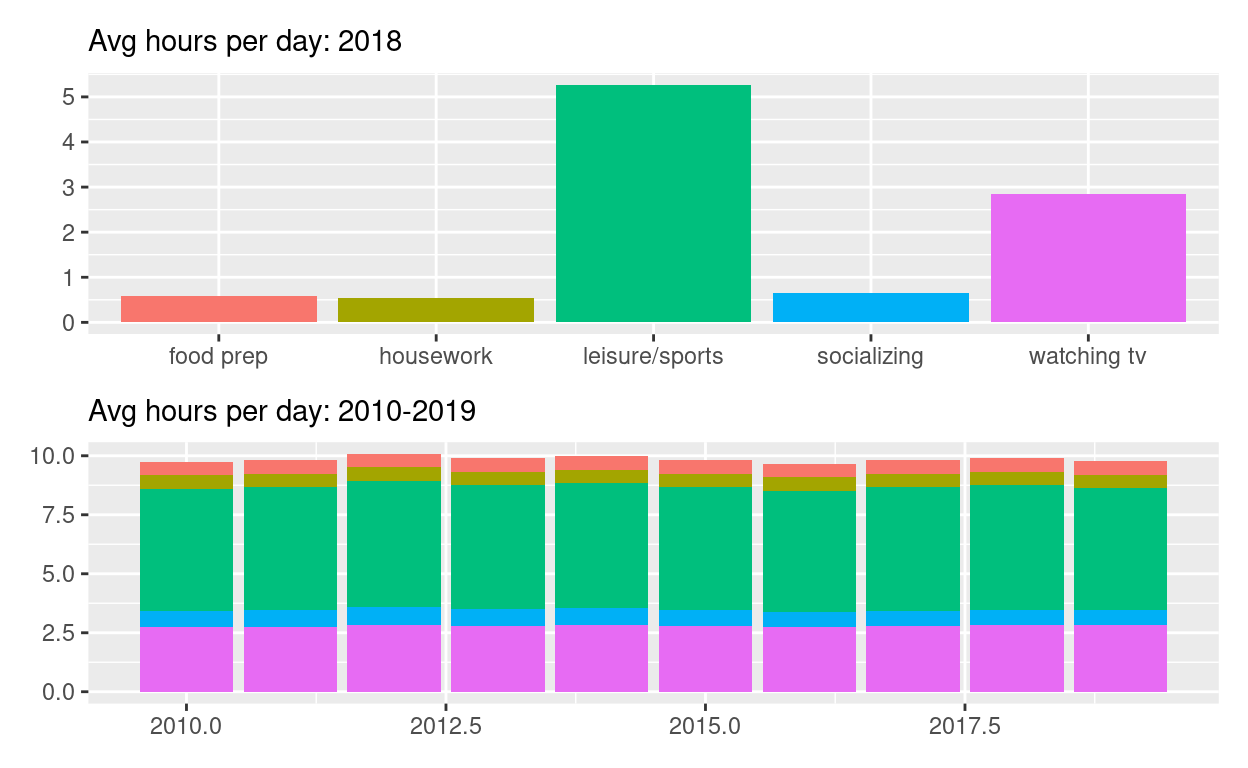Start with `p_all_no_legend`

`caption` to “Source: American Time of Use Survey, https://data.bls.gov/cgi-bin/surveymost?tu

``````p_all_no_legend  +
plot_annotation(title = "How much time Americans spent on selected activities",
caption = "Source: American Time of Use Survey, https://data.bls.gov/cgi-bin/surveymost?tu")
``````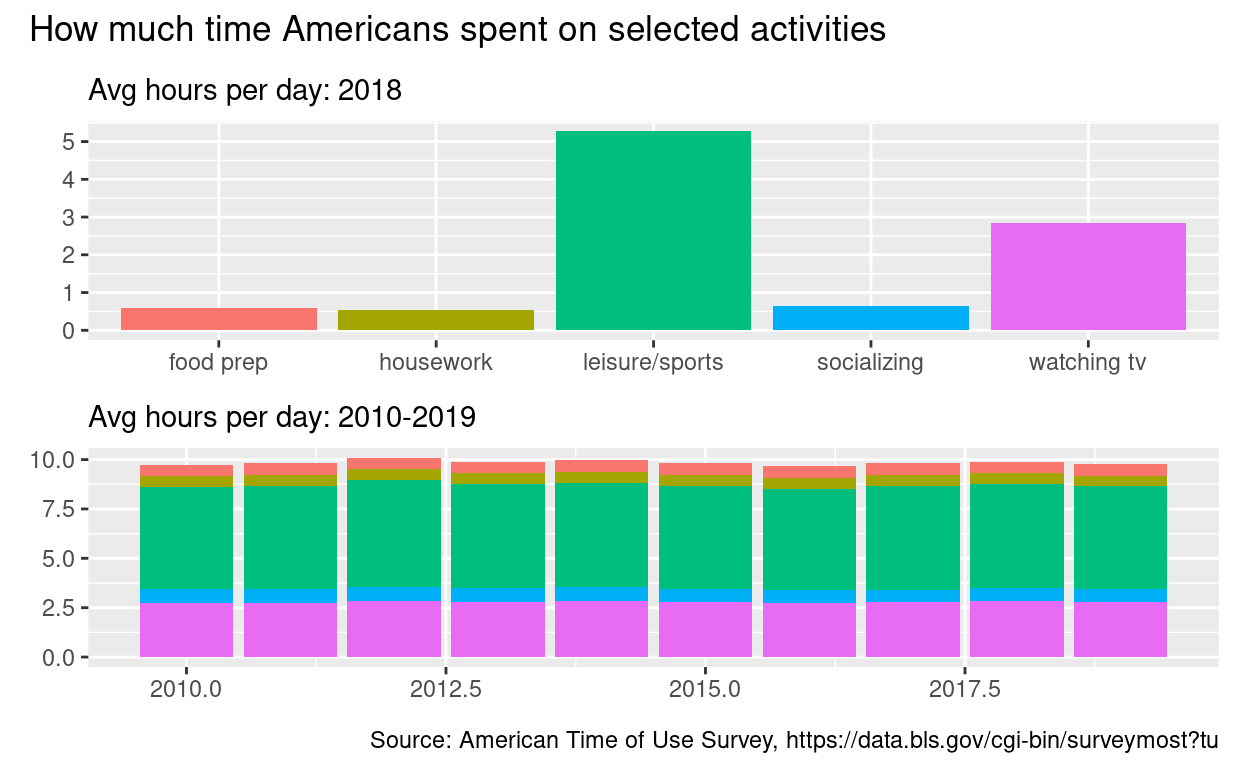# Question: Patchwork 2

`use`spend_time` from last question patchwork slides

Start with `spend_time`

• extract observations for food prep

• THEN create a plot with that data

• ADD points with `geom_point` – assign `year` to the x-axis

–assign `avg_hours` to the y-axis

-ADD line with `geom_smooth` –assign `year` to the x-axis

• assign `avg_hours` to the y-axis

• ADD breaks on for every year on x axis with with `scale_x_continuous`

• ADD `labs` to –set subtitle to Avg hours per day: food prep

– set x and y to NULL so x and y axes won’t be labeled

• assign the output to p4

• display p4

``````p4  <-
spend_time %>% filter(activity == "food prep")  %>%
ggplot() +
geom_point(aes(x = year, y = avg_hours)) +
geom_smooth(aes(x = year, y = avg_hours)) +
scale_x_continuous(breaks = seq(2010, 2019, by = 1)) +
labs(subtitle = "Avg hours per day: food prep", x = NULL, y = NULL)

p4
``````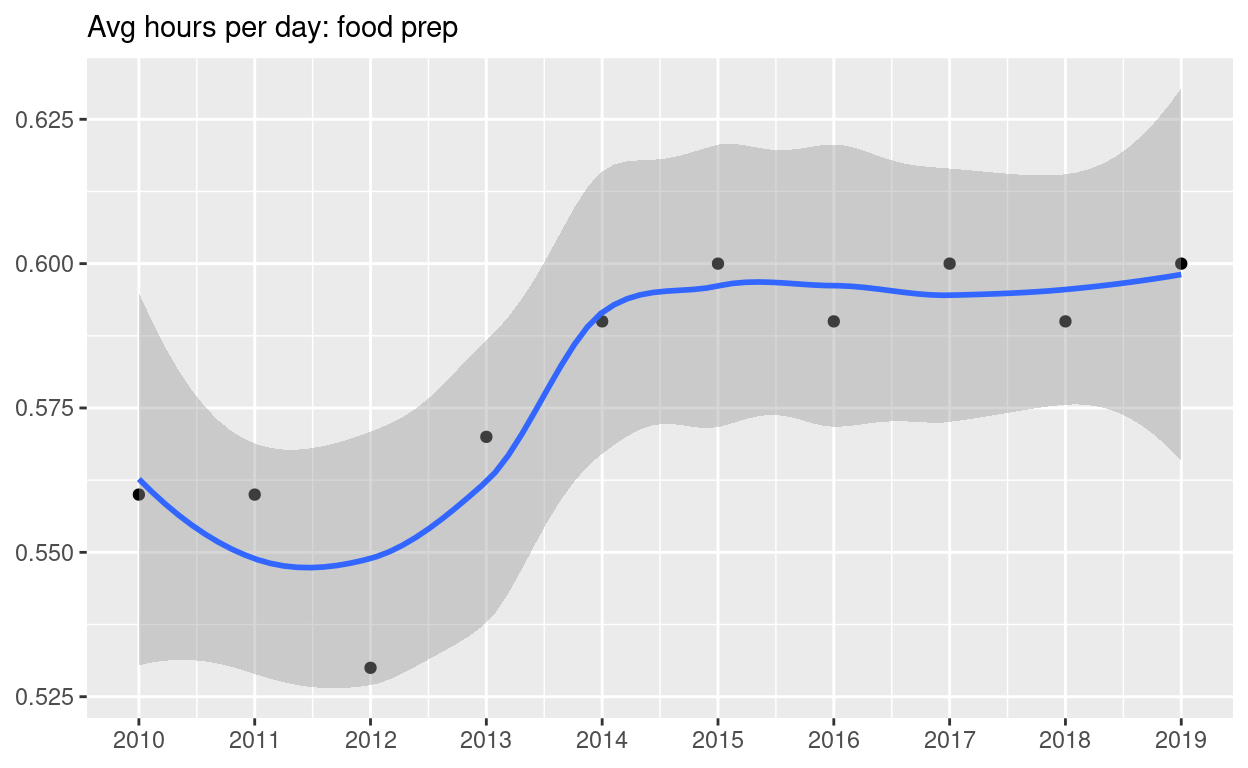• ADD `coord_cartesian` to change range on y axis to 0 to 6

• assign the output to `p5`

• display `p5`

``````p5 <-  p4 + coord_cartesian(ylim = c(0, 6))
p5
``````Start with `spend_time`

• create a plot with that data

• ADD points with `geom_point` –assign `year` to the x-axis

• assign `avg_hours` to the y-axis

• assign `activity` to color

• assign `activity` to group

• ADD line with `geom_smooth` – assign `year` to the x-axis

– assign `avg_hours` to the y-axis

– assign `activity` to color

– assign `activity` to group

• ADD breaks on for every year on x axis with with `scale_x_continuous`

• ADD `coord_cartesian` to change range on y axis to 0 to 6

• ADD `labs` to – set `x` and `y` to NULL so they won’t be labeled

• assign the output to `p6`

• display `p6`

``````p6   <-
spend_time  %>%
ggplot() +
geom_point(aes(x = year, y = avg_hours, color = activity, group = activity)) +
geom_smooth(aes(x = year, y = avg_hours, color = activity, group = activity)) +
scale_x_continuous(breaks = seq(2010, 2019, by = 1)) +
coord_cartesian(ylim = c(0, 6)) +
labs(x = NULL, y = NULL)

p6
``````Use patchwork to display `p4` and `p5` on top of `p6`

``````( p4 | p5 ) / p6
``````